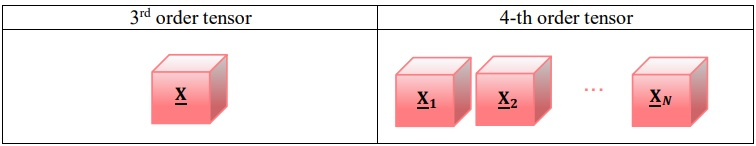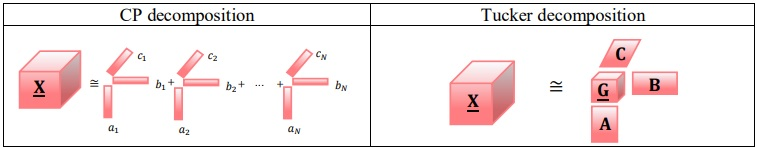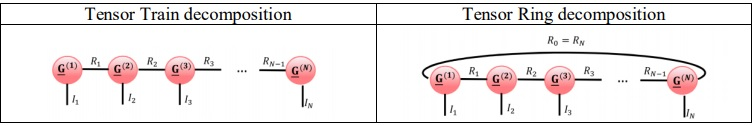x

## Tensor Analysis

The increasing scale and the volume of the acquired datasets form different physical phenomenon have made the tensors to be one of the important signal processing tools. Tensors are 3rd or higher order arrays which can be used for representing higher order datasets. An example for third and fourth order tensors have been illustrated in the following figures.Tensor decomposition is one of the main tensor analysis approaches, in which the original tensor has been decomposed into several smaller tensors and matrices. The resulting tensors and matrices after decomposition usually capture the characteristics of the original tensor and can be used in many applications such as tensor completion, tensor supper-resolution, tensor de-noising and … .

Several tensor decomposition approaches have been used in different signal processing applications. CP and Tucker decompositions are two well-known tensor decomposition approaches. The CP and Tucker decomposition for a 3rd order tensor have been illustrated in the following figures:CP and Tucker decompositions suffer from Curse of Dimensionality. This means that the volume of the resulting elements after decomposition, increases exponentially with the order of the original tensor, which makes a serious limitation for analyzing higher order tensors. So the researchers were motivated for introducing other tensor decomposition approaches in which the volume of the elements after decomposition, increases linearly by the order of the original tensor. These approaches construct a family of tensor decomposition methods, known as tensor networks. Two well-known members of this family are Tensor Train and Tensor Ring decompositions, illustrated in the following figures:The main aim of this research group is to use tensor networks, especially Tensor Train and Tensor Ring decompositions, for different biomedical applications, such as reconstruction, supper-resolution, de-noising, forecasting, … of different biomedical signals and images.